# Samacheer Kalvi 12th Maths Solutions Chapter 7 Applications of Differential Calculus Ex 7.3

## Tamilnadu Samacheer Kalvi 12th Maths Solutions Chapter 7 Applications of Differential Calculus Ex 7.3

Queston 1.
Explain why Rolle’s theorem is not applicable to the following functions in the respective intervals.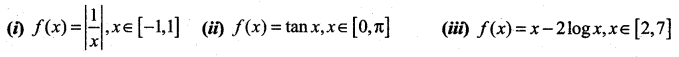Solution: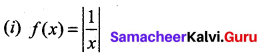f(x) is not continuous at x = 0. So Rolle’s Theorem is not applicable.

(ii) f (x) = tan x
f(x) is not continuous at x = $$\frac{\pi}{2}$$. So Rolle’s Theorem is not applicable..

(iii) f(x) = x – 2 log x
f(x) = x – 2 log x
f(2) = 2 – 2 log 2 = 2 – log 4
f(7) = 7 – 2 log 7 = 7 – log 49
f(2) ≠ f(7)
So Rolle’s theorem is not applicable.

Question 2.
Using the Rolle’s theorem, determine the values of x at which the tangent is parallel to the x-axis for the following functions: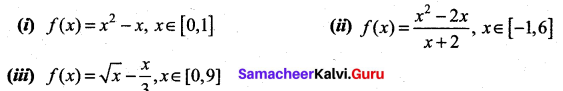Solution: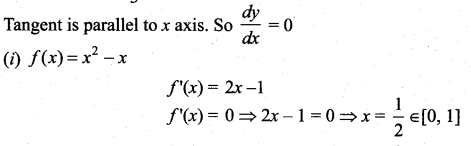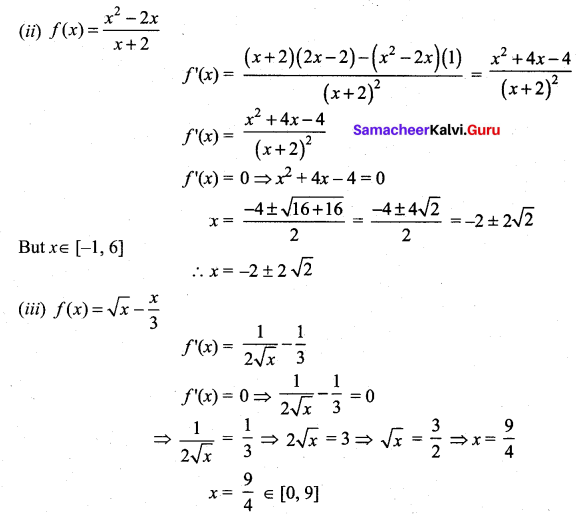Question 3.
Explain why Lagrange’s mean value theorem is not applicable to the following functions in the respective intervals :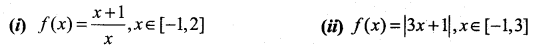Solution: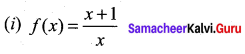The function is not continuous at x = 0. So Lagrange’s mean value theorem is not applicable in the given interval.

(ii) f(x) =|3x + 1|, x ∈ [-1, 3]
The function is not differentiable at x = $$\frac{-1}{3}$$. So Lagrange’s mean value theorem is not applicable in the given interval.

Question 4.
Using the Lagrange’s mean value theorem determine the values of x at which the tangent is parallel to the secant line at the end points of the given interval:
(i) f(x) = x3 – 3x + 2, x ∈ [-2, 2]
(ii) f(x) = (x – 2)(x – 7), x ∈ [3, 11]
Solution:
(i) f(x) = x3 – 3x + 2
Here a = -2, b = 2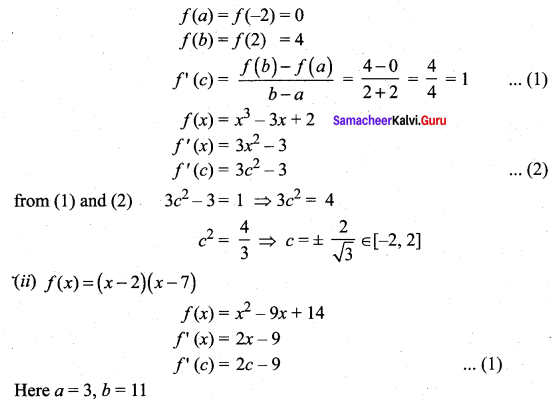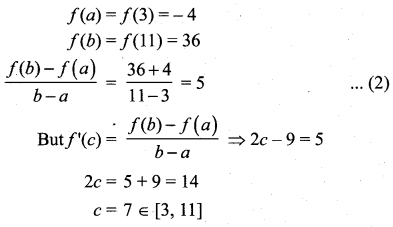Question 5.
Show that the value in the conclusion of the mean value theorem for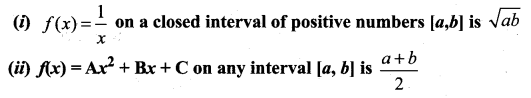Solution:
(i)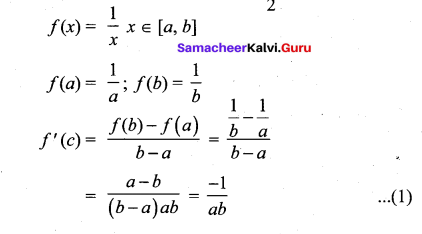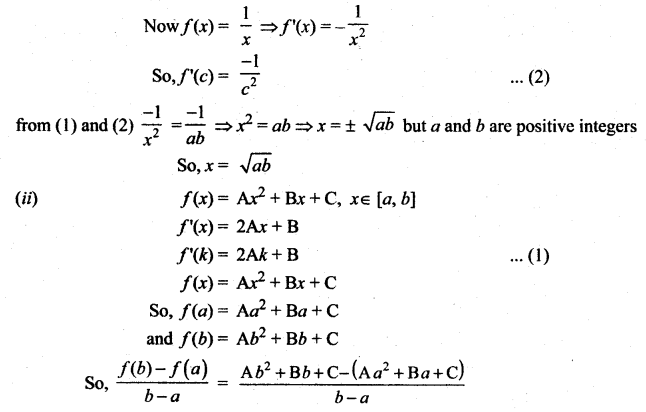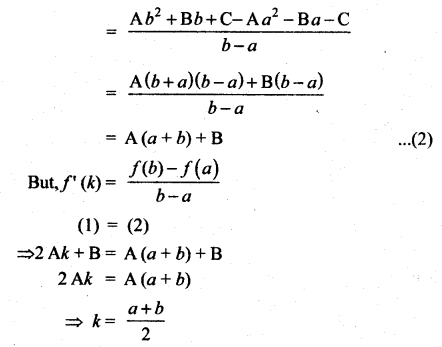Question 6.
A race car driver is racing at 20th km. If his speed never exceeds 150 km/hr, what is the maximum distance he can cover in the next two hours.
Solution: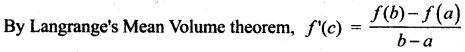Here the interval is [0, 2] and f(0) = 20, f(2) = ?
f(b) – f(a) ≤ (b – a)f’c)
here f (a) = 20
⇒ f(b) – 20 ≤ 150(2 – 0)
⇒ f(b) ≤ 320
(i.e) f(2) = 320 km.Question 7.
Suppose that for a function f(x), f'(x) ≤ 1 for all 1 ≤ x ≤ 4. Show that f(4) – f(1) ≤ 3.
Solution: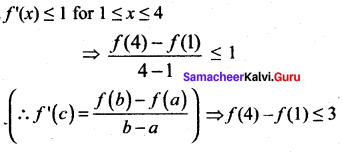Question 8.
Does there exist a differentiable function f(x) such that f(0) = -1, f(2) = 4 and f'(x) ≤ 2 for all x. Justify your answer.
Solution:
f(0) = -1, f(2) = 4, f(x) ≤ 2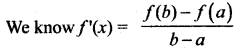Here a = 0, b = 2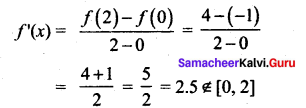So this is not possible

Question 9.
Show that there lies a point on the curve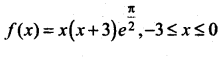where tangent drawn is parallel to the x – axis.
Solution: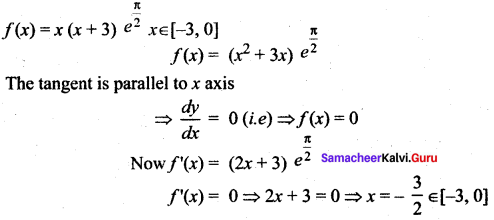⇒ There lies a point in [-3,0], where tangent is parallel to x axis.

Question 10.
Using mean value theorem prove that for, a > 0, b > 0, |e-a – e-b| < |a – b|
Solution: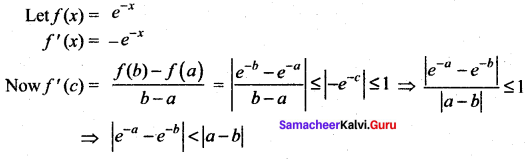### Samacheer Kalvi 12th Maths Solutions Chapter 7 Applications of Differential Calculus Ex 7.3 Additional Questions Solved

Question 1.
Verify Rolle’s theorem for the following:
(i) f(x) = x3 – 3x + 3, 0 ≤ x ≤ 1
(ii) f(x) = tan x, 0 ≤ x ≤ π
(iii) f(x) = | x |, – 1 ≤ x ≤ 1
(iv) f (x) = sin2 x, 0 ≤ x ≤ π
(v) f(x) = ex sin x, 0 ≤ x ≤ π
(vi) f(x) = x(x – 1) (x – 2), 0 ≤ x ≤ 2
Solution:
(i) f(x) = x3 – 3x + 3, 0 ≤ x ≤ 1. f is continuous on [0, 1] and differentiable in (0, 1)
f(0) = 3 and f(1) = 1 ∴ f(a) ≠ f(b)
∴ Rolle’s theorem, does not hold, since f(a) = f(b) is not satisfied.
Also note that f’ (x) = 3x2 – 3 = 0 ⇒ x2 = 1 ⇒ x = ±1
There exists no point c ∈ (0, 1) satisfying f’ (c) = 0.

(ii) f(x) = tan x, 0 ≤ x ≤ π.
f ‘(x) is not continuous in [0, π] as tan x tends to + ∞ at x = $$\frac{\pi}{2}$$,
∴ Rolle’s theorem is not applicable.

(iii) f (x) = | x |, -1 ≤ x ≤ 1
f is continuous in [-1, 1] but not differentiable in (-1, 1) since f'(0) does not exist.
∴ Rolle’s theorem is not applicable.

(iv) f (x) = sin2x, 0 ≤ x ≤ π
f is continuous in [0, π] and differentiable in (0, π). f(0) = f(π) = 0
(i.e.,) f satisfies hypothesis of Rolle’s theorem.
f’ (x) = 2 sin x cos x = sin 2x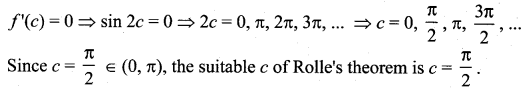(v) f(x) = ex, sin x, 0 ≤ x ≤ π
ex and sin x are continuous for all x, therefore the product ex sin x is continuous in 0 ≤ x ≤ π.
f’ (x) = ex sin x + ex cos x = ex (sin x + cos x) exist in 0 < x < π ⇒ f'(x) is differentiable in (0, π)
f (0) = e° sin 0 = 0
f (π) = eπ sin π = 0
∴ f satisfies hypothesis of Rolle’s theorem.
Thus there exists c ∈ (0, π) satisfying f'(c) = 0 ⇒ ec (sin c + cos c) = 0
⇒ ec = 0 or sin c + cos c = 0
⇒ ec = 0 ⇒ c = -∞ which is not meaningful here.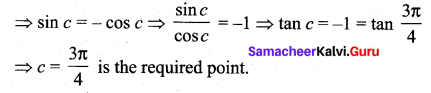(vi) f(x) = x (x – 1) (x – 2), 0 ≤ x ≤ 2
f is not continuous in [0, 2] and differentiable in (0, 2)
f(0) = 0 = f(2), satisfying hypothesis of Rolle’s theorem.
Now f'(x) = (x – 1) (x – 2) + x(x – 2) + x(x – 1) = 0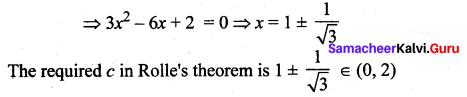Note: There could exist more than one such ‘c’ appearing in the statement of Rolle’s theorem.Question 2.
Suppose that f(0) = -3 and f'(x) ≤ 5 for all values of x, how large can f(2) possibly be?
Solution:
Since by hypothesis f is differentiable, f is continuous everywhere. We can apply Lagrange’s Law of the mean on the interval [0, 2], There exist atleast one ‘c’ ∈ (0, 2) such that
f(2) – f(0) = f'(c)(2 – 0)
f(2) = f(0) + 2f'(c) = -3 + 2f'(c)
Given that f'(x) ≤ 5 for all x.
In particular we know that f'(c) ≤ 5. Multiplying both sides of the inequality by 2, we have
2f'(c) ≤ 10
f(2) = -3 + 2f'(c) < -3 + 10 = 7
i.e., the largest possible value of f(2) is 7.

Question 3.
Using Rolle’s theorem find the points on the curves = x2 +1, -2 ≤ x ≤ 2 where the tangent is parallel to X-axis
Solution: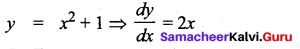a = -2,
b = 2
f(x) = x2 + 1
f(a) = f(-2) = 4 + 1 = 5
f(b) = f(2) = 4 + 1 = 5
So, f(a) = f(b)
f'(x) = 2x
f'(x) = 0 ⇒ 2x = 0
x = 0 where 0 ∈ (-2, 2)
at x = 0, y = 0 + 1 = 1
So, the point is (0, 1) at (0, 1) the tangent drawn is parallel to X-axis

Question 4.
Find ‘C’ of Lagrange’s mean value theorem for the function f(x) = 2x3 + x2 – x – 1, [0, 2]
Solution:
f(x) = 2x3 + x2 – x – 1
a = 0,
b = 2
f(a) = f(0) = -1
f(b) = f(2) = 2(8) = 4 – 2 – 1 = 16 + 4 – 2 – 1 = 17
By Lagrange’s mean value theorem, we get a constant c ∈ (a, b) such that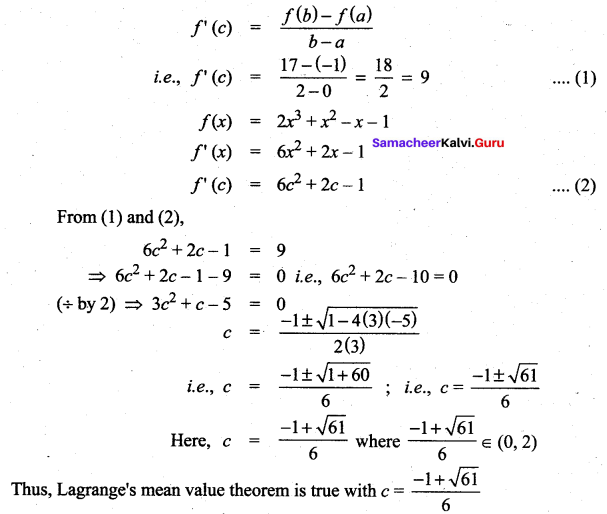Question 5.
Find ‘C’ of Lagrange’s mean value theorem for the function f(x) = x3 + x2 – 3x in [1, 3]
Solution:
f(x) = x3 + x2 – 3x
a = 1,
b=3
f(a) = f(1) = 1 – 5 – 3 = -7
f(b) = f(3) = 27 – 5(9) – 3(3)
= 27 – 45 – 9 = -27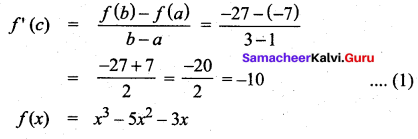f'(x) = 3x2 – 10x – 3
f'(x) = 3c2 – 10c – 3
From (1) and (2),
3c2 – 10c – 3 = -10
3c2 – 10c – 3 + 10 = 0
3c2 – 10c + 7 = 0
3c2 – 3c – 7c + 7 = 0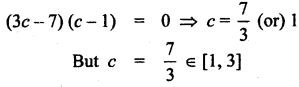So, Lagrange’s mean value theorem is true with c = $$\frac{7}{3}$$